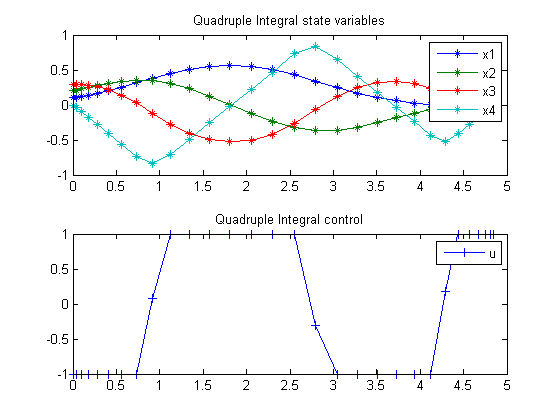ITERATIVE DYNAMIC PROGRAMMING, REIN LUUS

12.4.5 Example 5

CHAPMAN & HALL/CRC Monographs and Surveys in Pure and Applied Mathematics

89.1  Problem Formulation

Find u over t in [0; t_F ] to minimize

 J = tF

subject to:

 dx1 dt
= x2
 dx2 dt
= x3
 dx3 dt
= x4
 dx4 dt
= u

The initial condition are:

 x(0)  = [0.1  0.2  0.3  0]
 x(tF) = [0  0  0  0]
 −1 <= u <= 1

Reference: 

89.2  Problem setup

toms t
toms t_f
p = tomPhase('p', t, 0, t_f, 30);
setPhase(p);

tomStates x1 x2 x3 x4
tomControls u

% Initial guess
x0 = {t_f == 5
icollocate({x1 == 0.1-0.1*t/t_f
x2 == 0.2-0.2*t/t_f; x3 == 0.3-0.3*t/t_f})
collocate(u == -1)};

% Box constraints
cbox = {0.1 <= t_f <= 100
-1 <= collocate(u) <= 1};

% Boundary constraints
cbnd = {initial({x1 == 0.1; x2 == 0.2; x3 == 0.3; x4 == 0})
final({x1 == 0; x2 == 0; x3 == 0; x4 == 0})};

% ODEs and path constraints
ceq = collocate({
dot(x1) == x2; dot(x2) == x3
dot(x3) == x4; dot(x4) == u});

% Objective
objective = t_f;

89.3  Solve the problem

options = struct;
solution = ezsolve(objective, {cbox, cbnd, ceq}, x0, options);
t  = subs(collocate(t),solution);
x1 = subs(collocate(x1),solution);
x2 = subs(collocate(x2),solution);
x3 = subs(collocate(x3),solution);
x4 = subs(collocate(x4),solution);
u  = subs(collocate(u),solution);
Problem type appears to be: lpcon
Starting numeric solver
===== * * * =================================================================== * * *
TOMLAB - Tomlab Optimization Inc. Development license  999001. Valid to 2011-02-05
=====================================================================================
Problem: ---  1: Quadruple Integral             f_k       4.849598187644263100
sum(|constr|)      0.000000078353877264
f(x_k) + sum(|constr|)      4.849598265998140300
f(x_0)      5.000000000000000000

Solver: snopt.  EXIT=0.  INFORM=1.
SNOPT 7.2-5 NLP code
Optimality conditions satisfied

FuncEv    1 ConstrEv   58 ConJacEv   58 Iter   31 MinorIter  474
CPU time: 0.250000 sec. Elapsed time: 0.282000 sec.

89.4  Plot result

subplot(2,1,1)
plot(t,x1,'*-',t,x2,'*-',t,x3,'*-',t,x4,'*-');
legend('x1','x2','x3','x4');